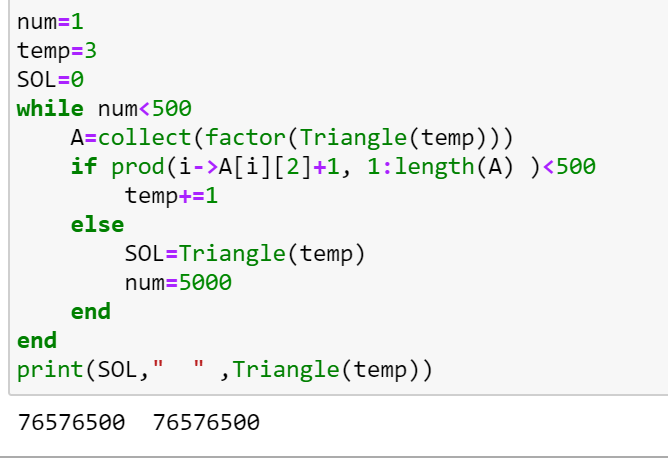# Strange output

using Primes

function Triangle(n)
return sum(1:n)
end

num=1
temp=3
while num<500

``````A=collect(factor(Triangle(temp)))
if prod(i->A[i]+1, 1:length(A) )<500
temp+=1
else
num=5000
ans=Triangle(temp)
end
``````

end
print(ans," " ,Triangle(temp))

I’m puzzled by why ans gives nothing, while its expected to be 76576500. Any explanation will be greatly appreciated.

I figured it out.
ans is probably one of the keywords in Julia.
So use a different name solves the problem.You can bring up help about `ans` by typing `?ans`:

``````help?> ans
search: ans transpose transcode expanduser instances MathConstants readlines

ans

A variable referring to the last computed value, automatically set at the
interactive prompt.
``````
1 Like